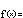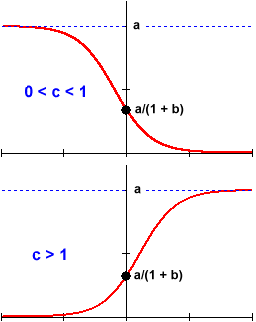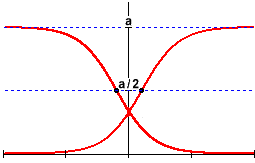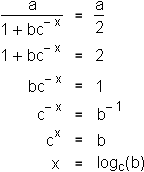Logistic Functions

Algebraic Representationf(x) = a / (1 + b c –x)

The algebra of the logistic family is something of a hybrid. It mixes together the behaviors of both exponentials and powers (proportions, like rational functions).

The study of logistic functions, therefore, begins to lead us away from the truly fundamental families of functions and into the larger world where descriptions of complex phenomena are composed of many functions. The logistic family's characteristic behavior appears often enough in applications, however, that it is worth examining in its own right.

The three parameters of the logistic family work together to produce its characteristic behavior, and they are best understood in combination.

The parameters  b  and  c  are simply the y-intercept and the base of the component exponential function  b c –x . As in other exponential functions, the base  c  is restricted to positive values. The only thing worth noting is the negative exponent, which inverts the typical dependence on the size of the base. Namely: While  c x  grows for  c > 1 ,  c –x  decays. Similarly, while  c x  decays for  0 < c < 1 ,  c –x  grows.The significance of the parameter  a  depends on the behavior of this exponential function. In the short term, when  x  is near  0  and  c –x  is near  1 , the value of the function is approximately  a/(1 + b) , regardless of the exponential's larger behavior. In the long term, however, it is important whether  c –x  grows or decays. If  c –x  grows (0 < c < 1), so does the denominator, and the function as a whole is driven to  0  in inverse proportion. If  c –x  decays (c > 1), the denominator approaches  1 , and the function as a whole grows to the value of the numerator: a .

It is this latter behavior, in which the function rises up to and eventually levels off at a constant horizontal asymptote, that is most often seen in models of "resource-limited" growth. Indeed, the function never exceeds the value  a . Thus, the parameter  a  is often called the limiting value or, in the description of populations, the carrying capacity.

The rate at which a logistic function falls from or rises to its limiting value is completely determined by the exponential function in the denominator. In particular, by the paramenters  b  and  c .

In the case of decay (0 < c < 1), the function first decreases at an increasing rate and then, half way down, begins to decrease at a decreasing rate. In other words, the curve changes from being concave down to concave up. Likewise for growth (c > 1): The function first increases at an increasing rate and then, half way up, begins to increase at a decreasing rate. That is, the curve changes from being concave up to concave down.

The change-over from a precipitous decrease or increase to the beginnings of an eventual levelling-off always occurs half-way up or down the logistic curve. This point of critical change in the function's behavior is called the inflection point.Using this information (which comes from calculus), we can calculate the inflection point's exact location: